Next: Time shifts and phase Up: Complex numbers Previous: Complex numbers   Contents   Index

## Complex sinusoids

Recall the formula for a (real-valued) sinusoid from Page: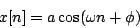This is a sequence of cosines of angles (called phases) which increase arithmetically with the sample number. The cosines are all adjusted by the factor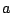. We can now rewrite this as the real part of a much simpler and easier to manipulate sequence of complex numbers, by using the properties of their arguments and magnitudes.Suppose that a complex numberhappens to have magnitude one and argument, so that it can be written as:Then for any integer, the numbermust have magnitude one as well (because magnitudes multiply) and argument(because arguments add). So,This is also true for negative values of, so for example,Figure 7.2 shows graphically how the powers ofwrap around the unit circle, which is the set of all complex numbers of magnitude one. They form a geometric sequence:and taking the real part of each term we get a real sinusoid with initial phase zero and amplitude one:Furthermore, suppose we multiply the elements of the sequence by some (complex) constantwith magnitudeand argument. This givesThe magnitudes are alland the argument of theth term is, so the sequence is equal to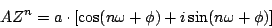and the real part is just the real-valued sinusoid:The complex numberencodes both the real amplitudeand the initial phase; the unit-magnitude complex numbercontrols the frequency which is just its argument.

Figure 7.2 also shows the sequence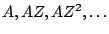; in effect this is the same sequence as, but amplified and rotated according to the amplitude and initial phase. In a complex sinusoid of this form,is called the complex amplitude.

Using complex numbers to represent the amplitudes and phases of sinusoids can clarify manipulations that otherwise might seem unmotivated. For instance, suppose we want to know the amplitude and phase of the sum of two sinusoids with the same frequency. In the language of this chapter, we let the two sinusoids be written as:whereand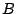encode the phases and amplitudes of the two signals. The sum is then equal to: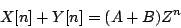which is a sinusoid whose amplitude equalsand whose phase equals. This is clearly a much easier way to manipulate amplitudes and phases than using properties of sines and cosines. Eventually, of course, we will take the real part of the result; this can usually be left to the end of whatever we're doing.Next: Time shifts and phase Up: Complex numbers Previous: Complex numbers   Contents   Index
Miller Puckette 2006-12-30